# ML | Implementing L1 and L2 regularization using Sklearn

Prerequisites: L2 and L1 regularization

This article aims to implement the L2 and L1 regularization for Linear regression using the Ridge and Lasso modules of the Sklearn library of Python.
Dataset – House prices dataset .

Step 1: Importing the required libraries

 `import` `pandas as pd ` `import` `numpy as np ` `import` `matplotlib.pyplot as plt ` `from` `sklearn.linear_model ``import` `LinearRegression, Ridge, Lasso ` `from` `sklearn.model_selection ``import` `train_test_split, cross_val_score ` `from` `statistics ``import` `mean `

 `# Changing the working location to the location of the data ` `cd C:\Users\Dev\Desktop\Kaggle\House Prices ` ` `  `# Loading the data into a Pandas DataFrame ` `data ``=` `pd.read_csv(``'kc_house_data.csv'``) ` ` `  `# Dropping the numerically non-sensical variables ` `dropColumns ``=` `[``'id'``, ``'date'``, ``'zipcode'``] ` `data ``=` `data.drop(dropColumns, axis ``=` `1``) ` ` `  `# Separating the dependent and independent variables ` `y ``=` `data[``'price'``] ` `X ``=` `data.drop(``'price'``, axis ``=` `1``) ` ` `  `# Dividing the data into training and testing set ` `X_train, X_test, y_train, y_test ``=` `train_test_split(X, y, test_size ``=` `0.25``) `

Step 3: Building and evaluating the different models

a) Linear Regression:

 `# Bulding and fitting the Linear Regression model ` `linearModel ``=` `LinearRegression() ` `linearModel.fit(X_train, y_train) ` ` `  `# Evaluating the Linear Regression model ` `print``(linearModel.score(X_test, y_test)) `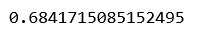b) Ridge(L2) Regression:

 `# List to maintain the different cross-validation scores ` `cross_val_scores_ridge ``=` `[] ` ` `  `# List to maintain the different values of alpha ` `alpha ``=` `[] ` ` `  `# Loop to compute the different values of cross-validation scores ` `for` `i ``in` `range``(``1``, ``9``): ` `    ``ridgeModel ``=` `Ridge(alpha ``=` `i ``*` `0.25``) ` `    ``ridgeModel.fit(X_train, y_train) ` `    ``scores ``=` `cross_val_score(ridgeModel, X, y, cv ``=` `10``) ` `    ``avg_cross_val_score ``=` `mean(scores)``*``100` `    ``cross_val_scores_ridge.append(avg_cross_val_score) ` `    ``alpha.append(i ``*` `0.25``) ` ` `  `# Loop to print the different values of cross-validation scores ` `for` `i ``in` `range``(``0``, ``len``(alpha)): ` `    ``print``(``str``(alpha[i])``+``' : '``+``str``(cross_val_scores_ridge[i])) `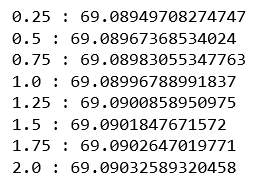From the above output, we can conclude that the best value of alpha for the data is 2.

 `# Building and fitting the Ridge Regression model ` `ridgeModelChosen ``=` `Ridge(alpha ``=` `2``) ` `ridgeModelChosen.fit(X_train, y_train) ` ` `  `# Evaluating the Ridge Regression model ` `print``(ridgeModelChosen.score(X_test, y_test)) `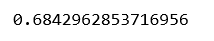c) Lasso(L1) Regression:

 `# List to maintain the cross-validation scores ` `cross_val_scores_lasso ``=` `[] ` ` `  `# List to maintain the different values of Lambda ` `Lambda ``=` `[] ` ` `  `# Loop to compute the cross-validation scores ` `for` `i ``in` `range``(``1``, ``9``): ` `    ``lassoModel ``=` `Lasso(alpha ``=` `i ``*` `0.25``, tol ``=` `0.0925``) ` `    ``lassoModel.fit(X_train, y_train) ` `    ``scores ``=` `cross_val_score(lassoModel, X, y, cv ``=` `10``) ` `    ``avg_cross_val_score ``=` `mean(scores)``*``100` `    ``cross_val_scores_lasso.append(avg_cross_val_score) ` `    ``Lambda.append(i ``*` `0.25``) ` ` `  `# Loop to print the different values of cross-validation scores ` `for` `i ``in` `range``(``0``, ``len``(alpha)): ` `    ``print``(``str``(alpha[i])``+``' : '``+``str``(cross_val_scores_lasso[i])) `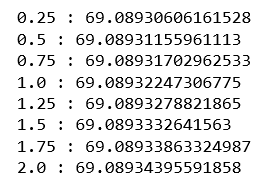From the above output, we can conclude that the best value of lambda is 2.

 `# Building and fitting the Lasso Regression Model ` `lassoModelChosen ``=` `Lasso(alpha ``=` `2``, tol ``=` `0.0925``) ` `lassoModelChosen.fit(X_train, y_train) ` ` `  `# Evaluating the Lasso Regression model ` `print``(lassoModelChosen.score(X_test, y_test)) `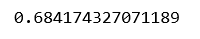Step 4: Comparing and Visualizing the results

 `# Building the two lists for visualization ` `models ``=` `[``'Linear Regression'``, ``'Ridge Regression'``, ``'Lasso Regression'``] ` `scores ``=` `[linearModel.score(X_test, y_test), ` `         ``ridgeModelChosen.score(X_test, y_test), ` `         ``lassoModelChosen.score(X_test, y_test)] ` ` `  `# Building the dictionary to compare the scores ` `mapping ``=` `{} ` `mapping[``'Linear Regreesion'``] ``=` `linearModel.score(X_test, y_test) ` `mapping[``'Ridge Regreesion'``] ``=` `ridgeModelChosen.score(X_test, y_test) ` `mapping[``'Lasso Regression'``] ``=` `lassoModelChosen.score(X_test, y_test) ` ` `  `# Printing the scores for different models ` `for` `key, val ``in` `mapping.items(): ` `    ``print``(``str``(key)``+``' : '``+``str``(val)) `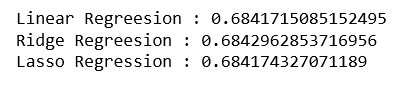`# Plotting the scores ` `plt.bar(models, scores) ` `plt.xlabel(``'Regression Models'``) ` `plt.ylabel(``'Score'``) ` `plt.show() `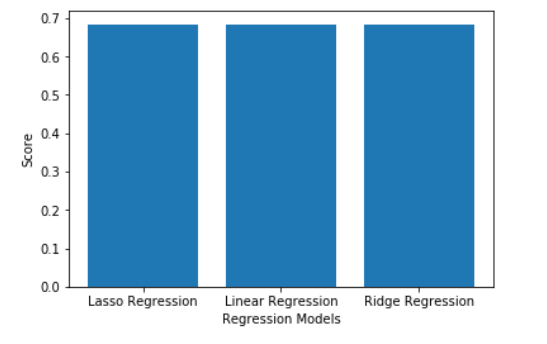My Personal Notes arrow_drop_upCheck out this Author's contributed articles.

If you like GeeksforGeeks and would like to contribute, you can also write an article using contribute.geeksforgeeks.org or mail your article to contribute@geeksforgeeks.org. See your article appearing on the GeeksforGeeks main page and help other Geeks.

Please Improve this article if you find anything incorrect by clicking on the "Improve Article" button below.

Improved By : shubham_singh

Article Tags :
Practice Tags :

Be the First to upvote.

Please write to us at contribute@geeksforgeeks.org to report any issue with the above content.